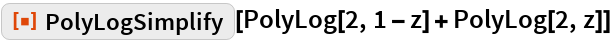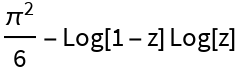#Function Repository Resource:

# PolyLogSimplify

Simplify polylogarithms in an expression

Contributed by: Wolfram Research
 ResourceFunction["PolyLogSimplify"][expr] transforms polylogarithm functions in expr, trying to either decrease the number of polylogarithm functions or convert combinations of them into more elementary functions.

## Details and Options

A function that is essentially equivalent to ResourceFunction["PolyLogSimplify"] is used inside FullSimplify and FunctionExpand.

## Examples

### Basic Examples

Simplify an expression involving polylogarithms:

 In:=Out=## Requirements

Wolfram Language 11.3 (March 2018) or above